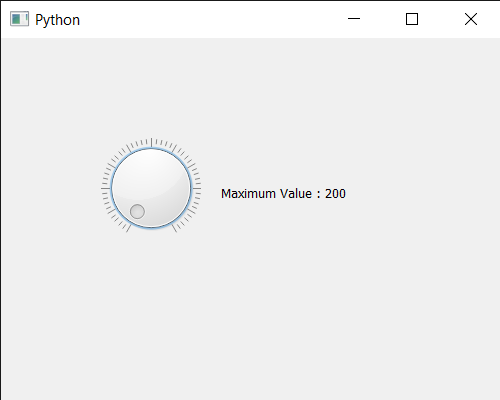# PyQt5 QDial – Getting Upper Bound

In this article we will see how we can get the upper bound of QDial. Upper bound means the highest value QDial can handle by default the maximum value is 99 although we can change it any time with the help of `setMaximum` method. With the upper bound we can make sure the number above the upper bound can be assigned. Setting upper bound will not effect the movement of the Qdial it will still able to move 360 degree.

In order to do this we use `maximum` method with the QDial object

Syntax : dial.maximum()

Argument : It takes no argument

Return : It returns integer

Below is the implementation

 `# importing libraries ` `from` `PyQt5.QtWidgets ``import` `*`  `from` `PyQt5 ``import` `QtCore, QtGui ` `from` `PyQt5.QtGui ``import` `*`  `from` `PyQt5.QtCore ``import` `*`  `import` `sys ` ` `  ` `  `class` `Window(QMainWindow): ` ` `  `    ``def` `__init__(``self``): ` `        ``super``().__init__() ` ` `  `        ``# setting title ` `        ``self``.setWindowTitle(``"Python "``) ` ` `  `        ``# setting geometry ` `        ``self``.setGeometry(``100``, ``100``, ``500``, ``400``) ` ` `  `        ``# calling method ` `        ``self``.UiComponents() ` ` `  `        ``# showing all the widgets ` `        ``self``.show() ` ` `  `    ``# method for components ` `    ``def` `UiComponents(``self``): ` ` `  `        ``# creating QDial object ` `        ``dial ``=` `QDial(``self``) ` ` `  `        ``# setting geometry to the dial ` `        ``dial.setGeometry(``100``, ``100``, ``100``, ``100``) ` ` `  `        ``# setting maximum value to the dial ` `        ``dial.setMaximum(``200``) ` ` `  `        ``# making notch visible ` `        ``dial.setNotchesVisible(``True``) ` ` `  ` `  `        ``# creating a label ` `        ``label ``=` `QLabel(``"GeeksforGeeks"``, ``self``) ` ` `  `        ``# setting geometry to the label ` `        ``label.setGeometry(``220``, ``125``, ``200``, ``60``) ` ` `  `        ``# making label multiline ` `        ``label.setWordWrap(``True``) ` ` `  `        ``# adding action to the dial ` `        ``dial.valueChanged.connect(``lambda``: label.setText(``"Value = "` `+` `str``(dial.value()))) ` ` `  `        ``# getting maximum value ` `        ``value ``=` `dial.maximum() ` ` `  `        ``# setting text to the label ` `        ``label.setText(``"Maximum Value : "` `+` `str``(value)) ` `         `  ` `  ` `  `# create pyqt5 app ` `App ``=` `QApplication(sys.argv) ` ` `  `# create the instance of our Window ` `window ``=` `Window() ` ` `  `# start the app ` `sys.exit(App.``exec``()) `

Output :Attention geek! Strengthen your foundations with the Python Programming Foundation Course and learn the basics.

To begin with, your interview preparations Enhance your Data Structures concepts with the Python DS Course.

My Personal Notes arrow_drop_upCheck out this Author's contributed articles.

If you like GeeksforGeeks and would like to contribute, you can also write an article using contribute.geeksforgeeks.org or mail your article to contribute@geeksforgeeks.org. See your article appearing on the GeeksforGeeks main page and help other Geeks.

Please Improve this article if you find anything incorrect by clicking on the "Improve Article" button below.

Article Tags :

Be the First to upvote.

Please write to us at contribute@geeksforgeeks.org to report any issue with the above content.